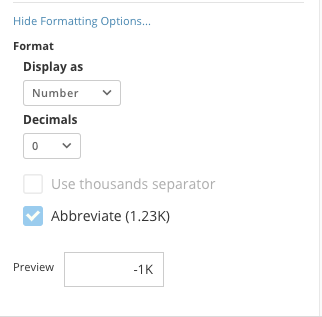# I have a card that displays Total Tasks and Performance per Task (Minutes).

When I use Beast Mode to modify the Summary Number, the I receive an error "unable to load at this time".

My Performance per Task (Minutes) Beast Mode due to TotalTaskTime is decimal.

My Minutes per Task Beast Mode which displays valid formula.

An example is Total Tasks 4 and Performance per Task (Minutes) 44 thus the Minutes per Task would be 11 and that is what I would want the Summary Number to display.

Other factors are this card is a table with subtotals by user.  I have attached a screen print for review.

Michael

•

Domo doesn't like your SUM(SUM()) without a windowing function which is why it's throwing an error.

If you're looking just for minutes per task for the overall dataset you could do:

`SUM(`TotalTaskTime`*60)/SUM(`TotalTasks`)`
**Did this solve your problem? Accept it as a solution!**
• @GuitarMan2020 - You're close. ROUND is a function so it'd look like this (assuming 1 digit after the decimal)

`ROUND(SUM(`TotalTaskTime`*60)/SUM(`TotalTasks`), 1)`

A better way though would be to utilize the formatting options (blue link at the bottom of the summary number window to expand) under the Summary Number and set the decimals to 1 there instead and make sure it's displaying as a number.**Did this solve your problem? Accept it as a solution!**

• Excellent guidance!  One last question is how would I round the Summary Number?  It is currently displaying about 16 places from the decimal point.  Would it be this -